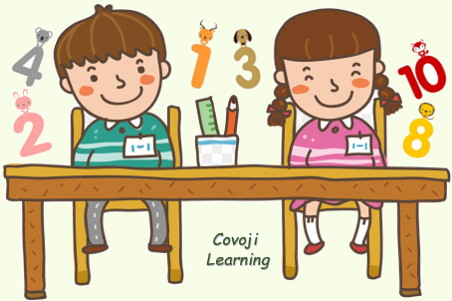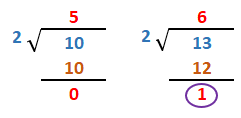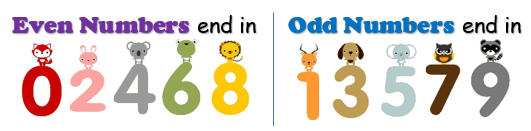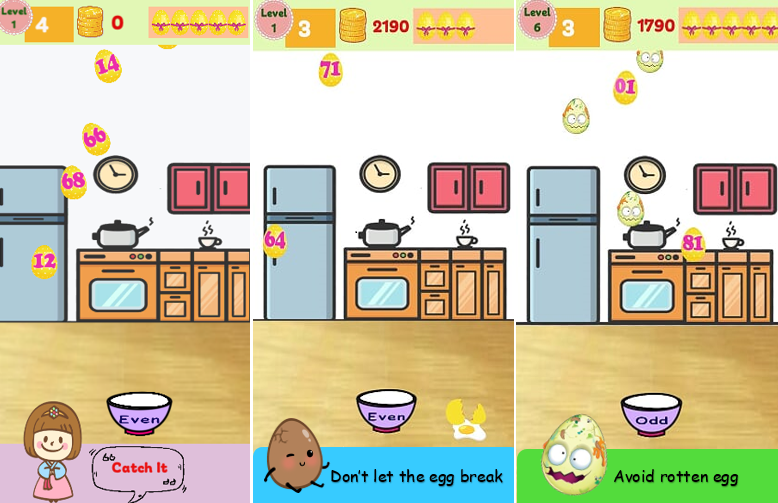Even and Odd Numbers

Even numbers are numbers that can be divided evenly by 2. Odd numbers are numbers that cannot be divided evenly by 2.Even and Odd numbers can be shown as:When you teach kids to identify larger odd and even numbers,  instructing them to look at the ones place. That single number will tell you whether the entire number is odd or even. For example, the number 37. It ends in 7, an odd number.

Egg Even Odd is the game that helps kids to distinguish between odd and even numbers, let’s extend the learning idea with fun game to help kids to understand this concept quickly!Categories: Math Zone